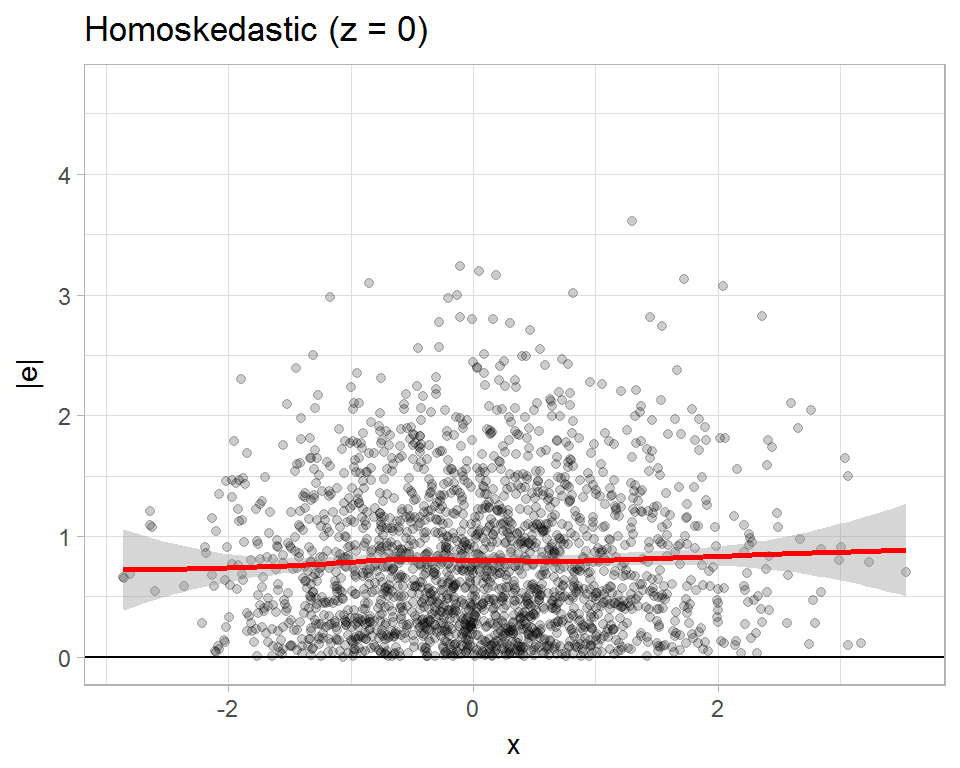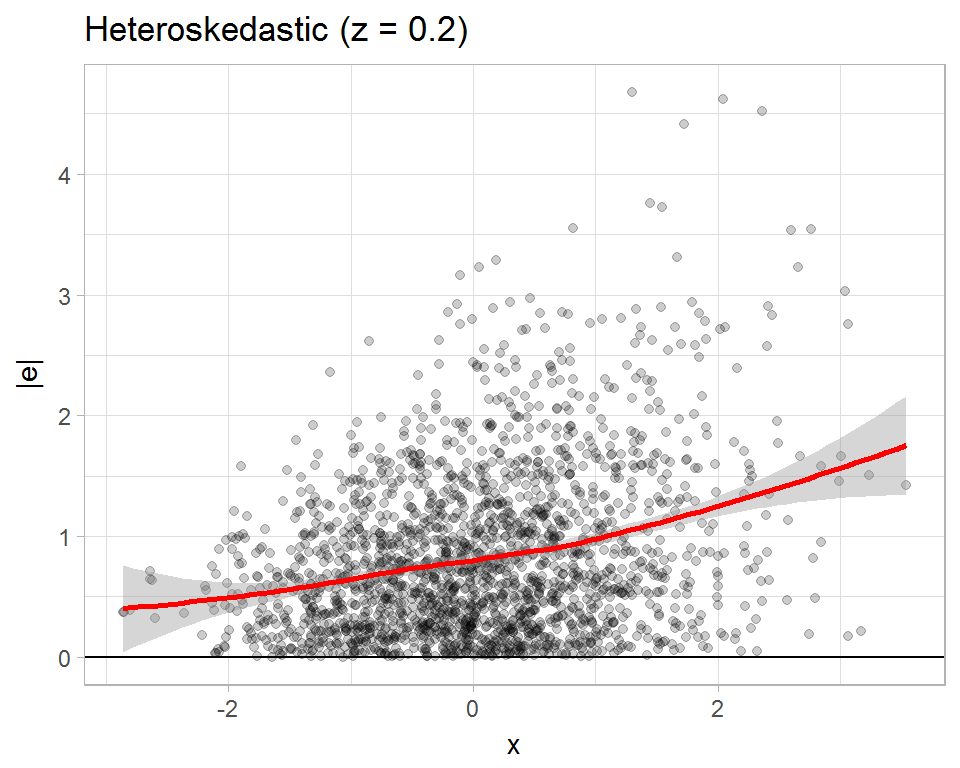4/30/2017

# Linear regression

Let’s talk about a basic regression model:

\begin{aligned} y_i &= \beta_0 + \beta_1 x_{1i} + \cdots + \beta_{p-1} x_{p-1,i} + e_i \\ \mathbf{y} &= \mathbf{X} \boldsymbol\beta + \mathbf{e} \end{aligned} estimated by ordinary least squares:

$\boldsymbol{\hat\beta} = \left(\mathbf{X}'\mathbf{X}\right)^{-1} \mathbf{X}'\mathbf{y}.$

• Classical inference methods assume homoskedasticity: $$\text{Var}(e_i) = \sigma^2$$
• But there are many settings where the we would rather allow for heteroskedasticity of an unknown form: $$\text{Var}(e_i) = \sigma_i^2$$
• One way to do inference in this setting is by using heteroskedasticity-consistent covariance matrix estimators (HCCMEs; Eicker, 1967; Huber, 1967; White, 1980).

# HCCMEs

• Variance of the OLS estimator: $\text{Var}\left(\mathbf{c}'\boldsymbol{\hat\beta}\right) = \frac{1}{n} \mathbf{c}'\mathbf{M} \left(\frac{1}{n}\sum_{i=1}^n \sigma_i^2 \mathbf{x}_i\mathbf{x}_i'\right) \mathbf{M}\mathbf{c}, \qquad \mathbf{M} = \left(\frac{1}{n}\mathbf{X}'\mathbf{X}\right)^{-1}$
• The original HCCME (sandwich) estimator: $\mathbf{V}^{HC0} = \frac{1}{n} \mathbf{c}'\mathbf{M} \left(\frac{1}{n}\sum_{i=1}^n \hat{e}_i^2 \mathbf{x}_i\mathbf{x}_i'\right) \mathbf{M}\mathbf{c}$
• HC0 is asymptotically consistent, but biased in small samples; hypothesis tests based on HC0 can have poor coverage in small samples.
• Features of design matrix (especially leverage) influence bias and coverage.

# Potential small-sample improvements

• Modified sandwich estimators (MacKinnon & White, 1985; Davidson & MacKinnon, 1993): $\mathbf{V}^{HC0} = \frac{1}{n} \mathbf{c}'\mathbf{M} \left(\frac{1}{n}\sum_{i=1}^n \color{red}{\omega_i} \hat{e}_i^2 \mathbf{x}_i\mathbf{x}_i'\right) \mathbf{M}\mathbf{c}$ where \small \begin{aligned} \text{HC1:} \qquad \omega_i &= n / (n - p) \\ \text{HC2:} \qquad \omega_i &= (1 - h_{ii})^{-1} \\ \text{HC3:} \qquad \omega_i &= (1 - h_{ii})^{-2} \end{aligned}
• Long and Ervin (2000) conducted a comprehensive simulation study on hypothesis test coverage with HCCMEs, recommended HC3 as default.
• Subsequently (Cribari-Neto et al., 2004, 2007, 2011): \small \begin{aligned} \text{HC4:} \qquad \omega_i &= (1 - h_{ii})^{-\delta_i}, \qquad \delta_i = \min\{h_{ii} n / p, 4\} \\ \text{HC4m:} \qquad \omega_i &= (1 - h_{ii})^{-\delta_i}, \qquad \delta_i = \min\left\{h_{ii} n / p, 1 \right\} + \min\left\{h_{ii} n / p, 1.5 \right\} \\ \text{HC5:} \qquad \omega_i &= (1 - h_{ii})^{-\delta_i}, \qquad \delta_i = \min\left\{h_{ii} n / p, \max \left\{4, 0.7 h_{(n)(n)} n / p\right\}\right\} \end{aligned}

# Other potential small-sample improvements

Approximations for the reference distribution of test statistic:

• Satterthwaite approximation (Lipsitz, Ibrahim, & Parzen, 1999)
• Edgeworth approximation (Kauermann & Carroll, 2001)
• Saddlepoint approximation (McCaffrey & Bell, 2006)
• All three approximations developed assuming a homoskedastic “working model” for the error structure.
• These approaches have been largely ignored. Never compared to HC* variants or to each other.

# Simulations

• Simple regression with one predictor: $y_i = \beta_0 + \beta_1 x_i + \sigma_i e_i$
• Predictor variable $$x_i \sim \chi^2$$ with varying degrees of skewness
• Errors $$e_i \sim N(0, 1)$$, $$t_5$$, or $$\chi^2_5$$
• Skedasticity function $$\sigma_i = \exp(\zeta x_i)$$, $$\zeta = 0.00,0.02,0.04,...,0.20$$
• sample sizes of $$n = 25, 50, 100$$
• Target Type-I error rates of $$\alpha = .05, .01, .005$$
• Rejection rates estimated from 50000 replications

# Size of HC* variants, $$\alpha = .05$$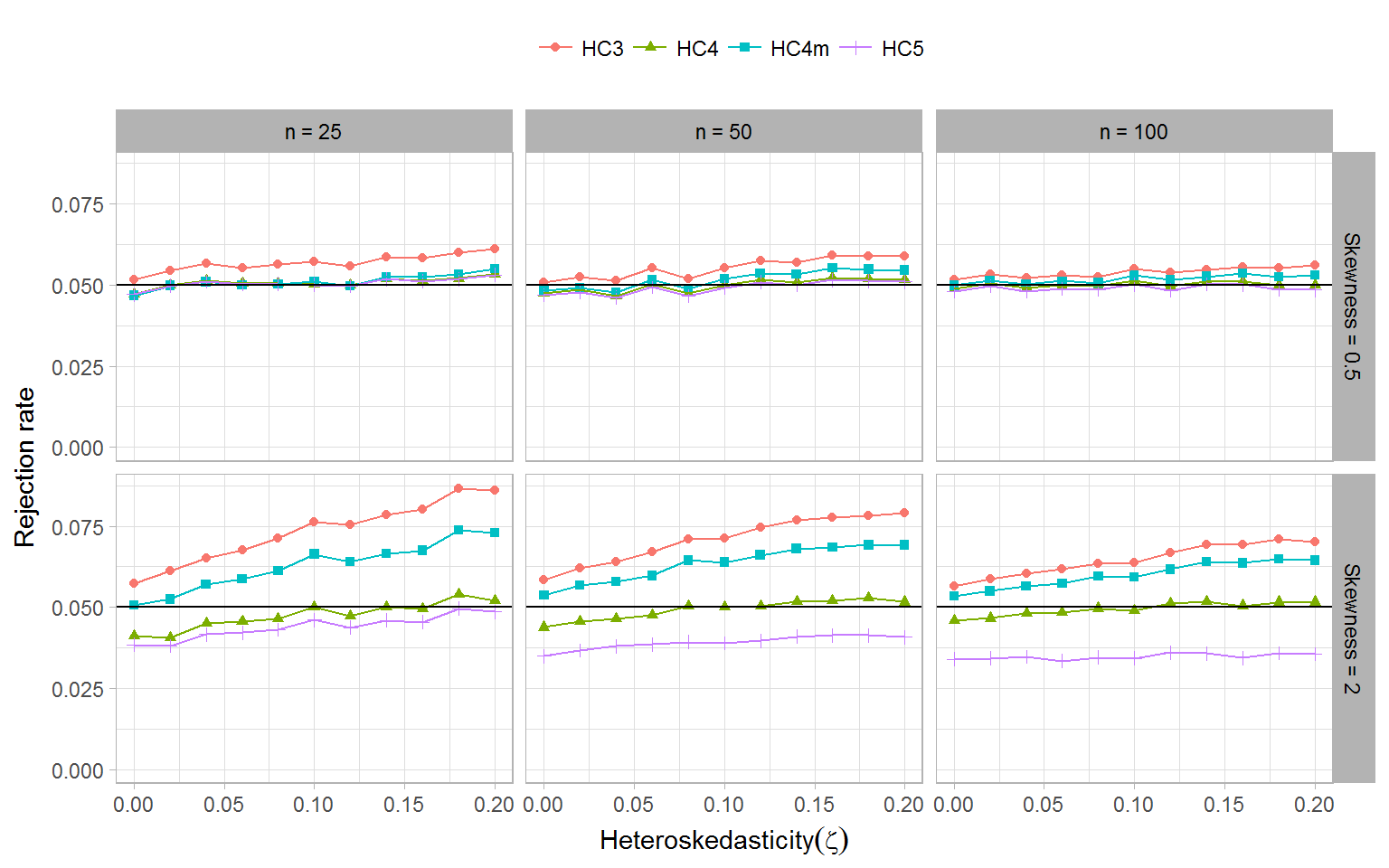# Size of HC* variants, $$\alpha = .01$$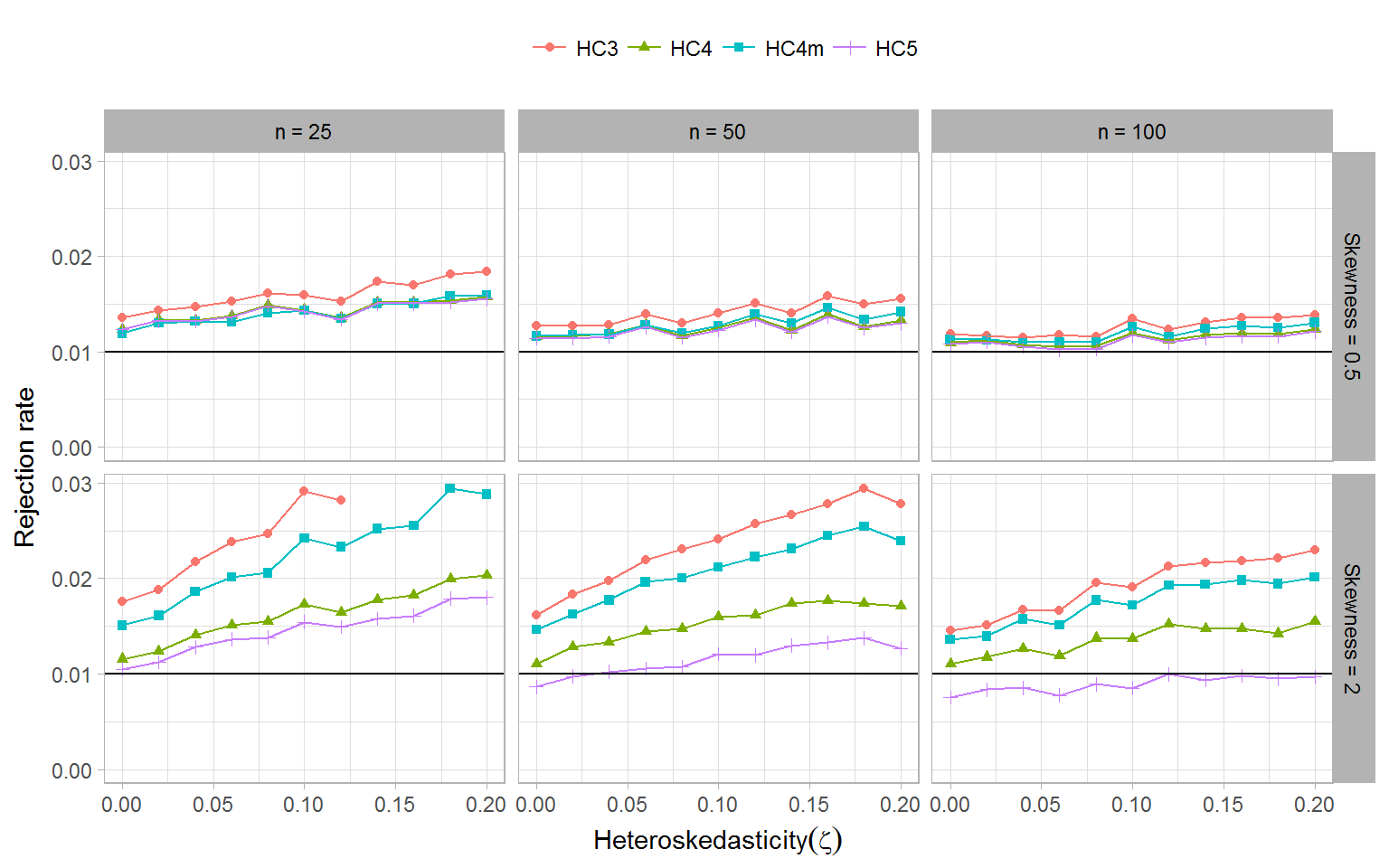# Size of HC* variants, $$\alpha = .005$$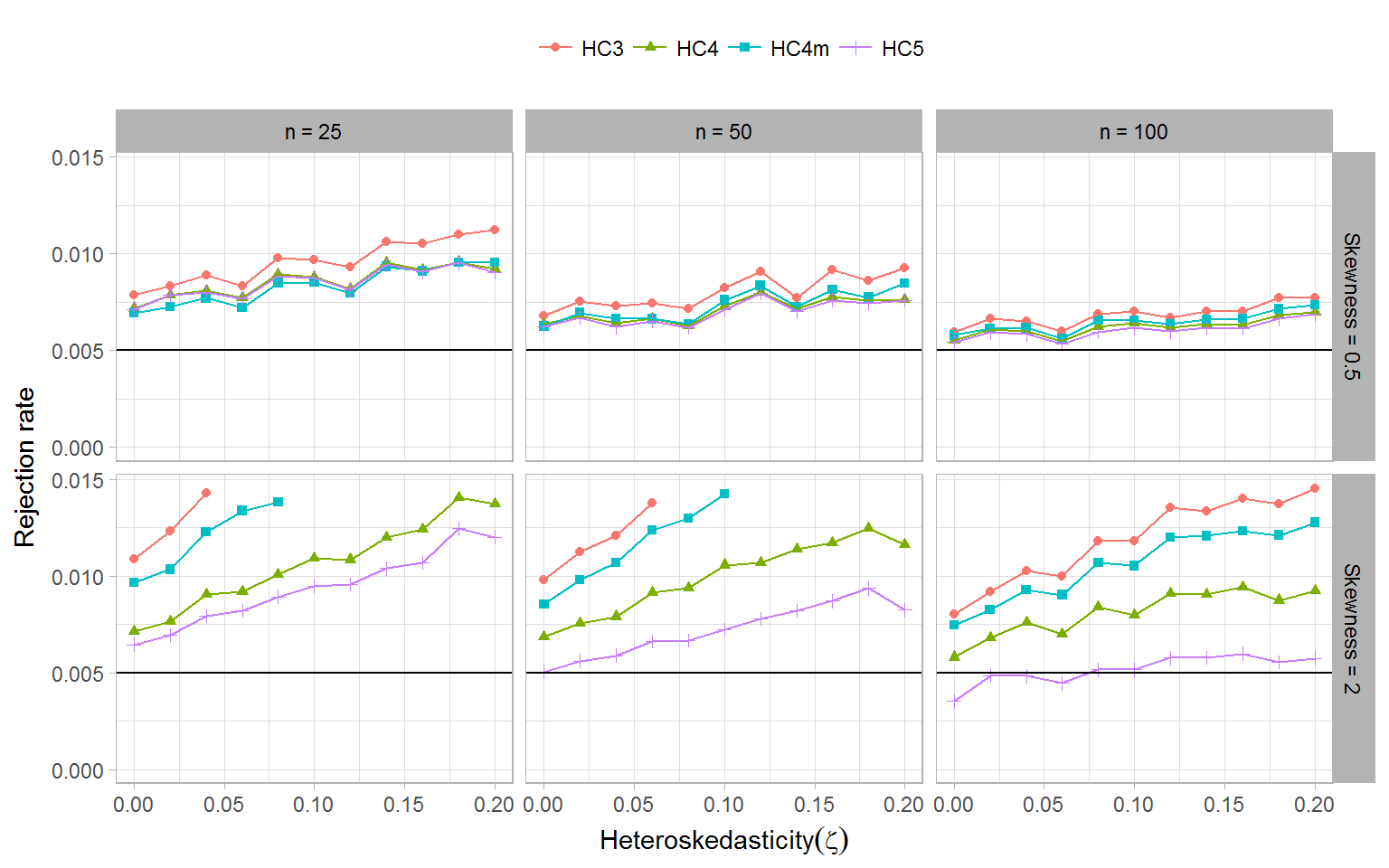# Size of selected tests, $$\alpha = .05$$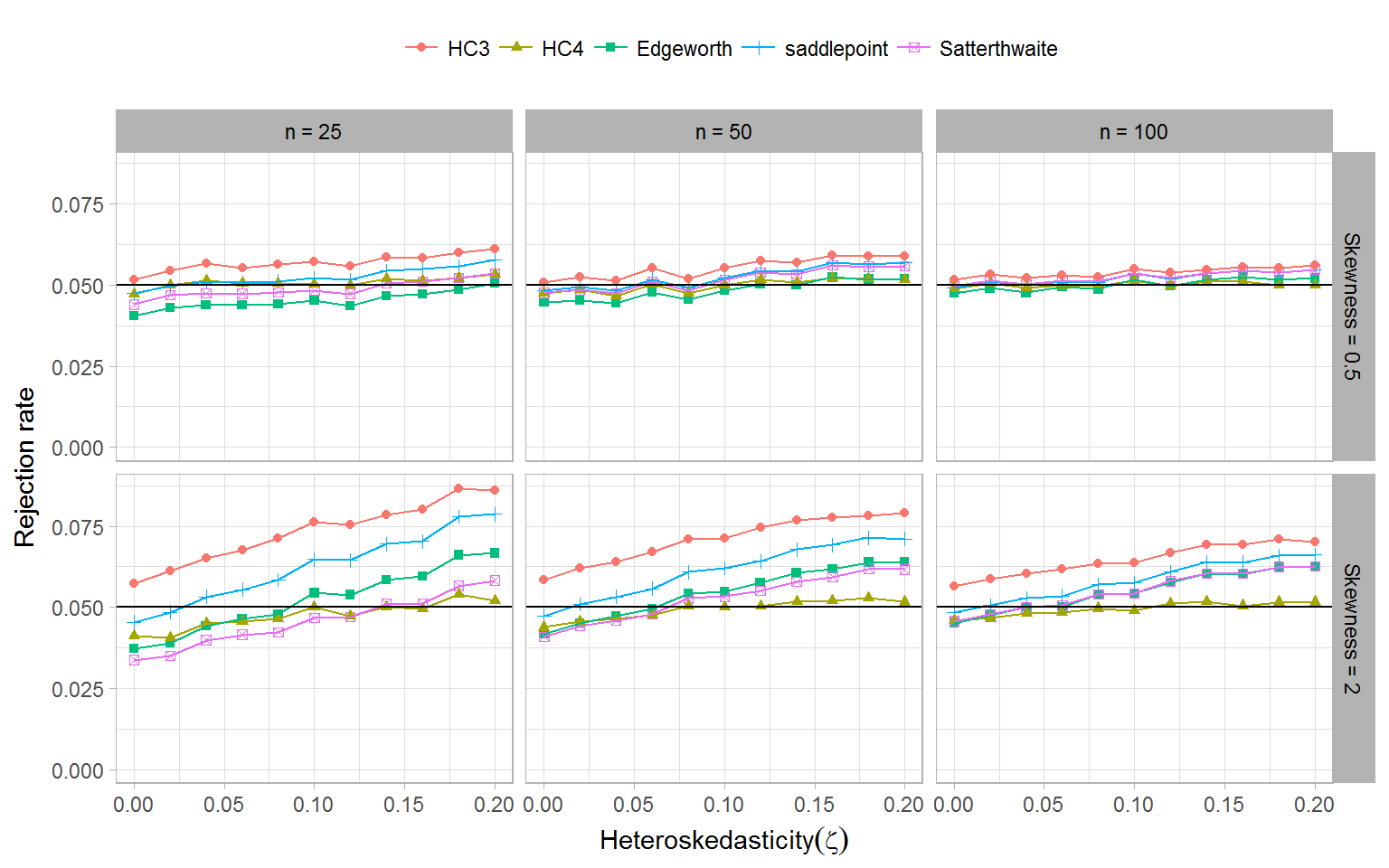# Size of selected tests, $$\alpha = .01$$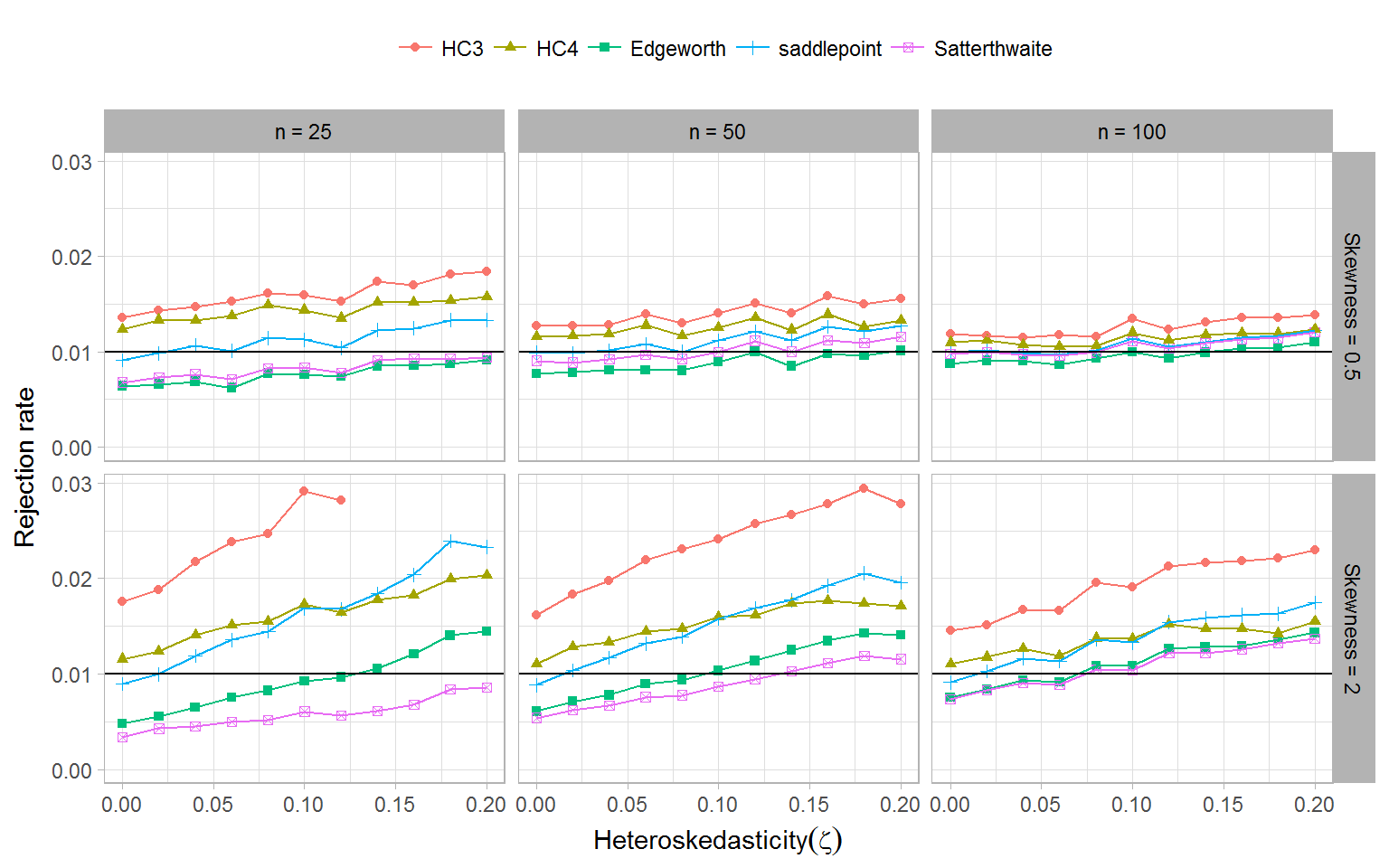# Size of selected tests, $$\alpha = .005$$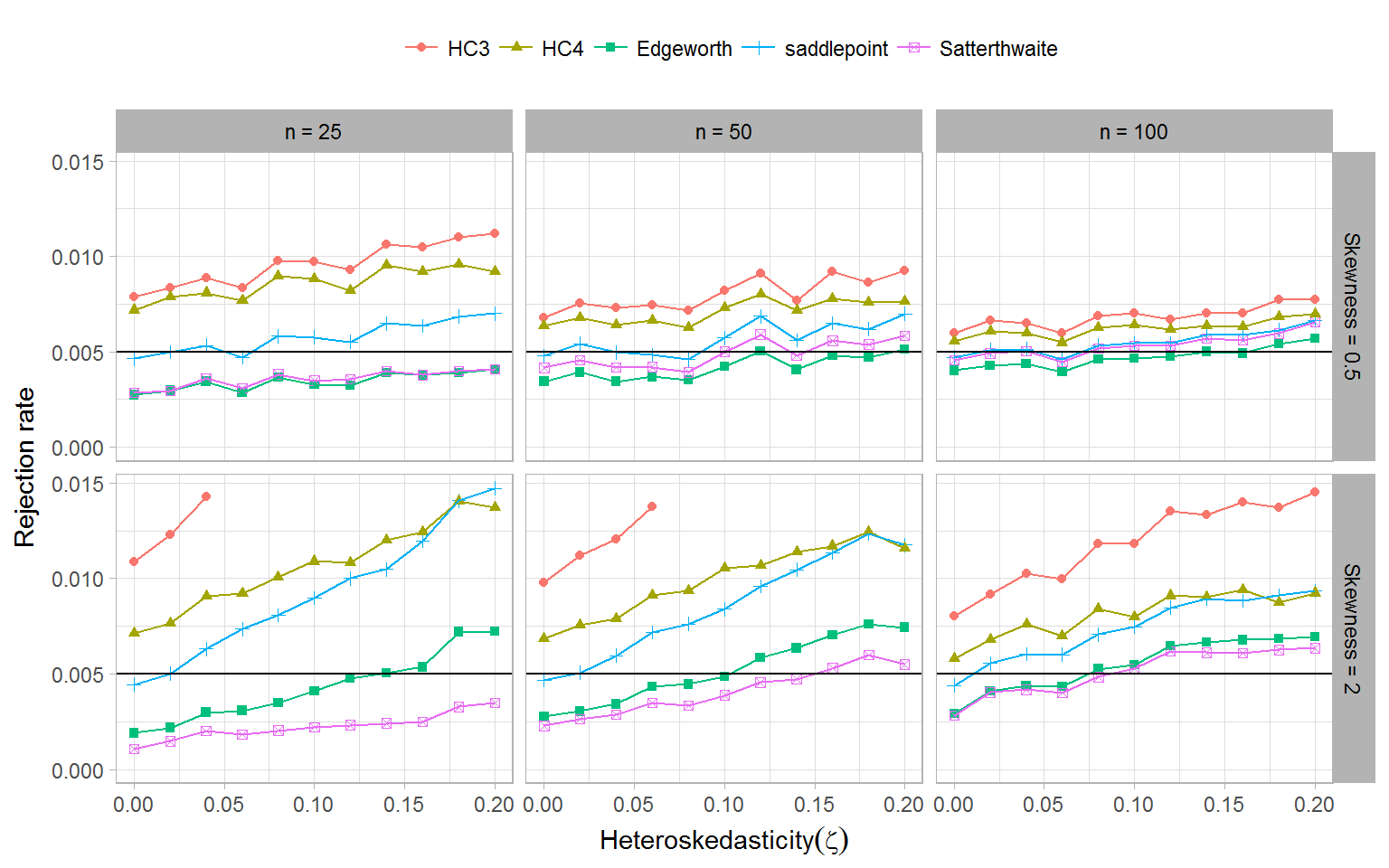# Findings

1. Currently recommended test HC3 does not adequately control type-I error rate.
2. At the $$\alpha = .05$$ level, HC4 maintains most accurate rejection rates of all tests considered.
3. At smaller $$\alpha$$ levels, Satterthwaite and Edgeworth approximations out-perform HC3 and HC4.

# Discussion

• In ongoing work, we are examining the relative power of tests (after size adjustment)
• Generality of findings requires further investigation (covariate distribution, skedasticity function, number of predictors)
• Distributional approximations warrant wider consideration because they can be generalized to more complex models.

# Degree of heteroskedasticity